## Artigo

•Citado por SciELO
•Acessos

•Citado por Google
•Similares em SciELO
•Similares em Google

## versão impressa ISSN 0122-5383

### C.T.F Cienc. Tecnol. Futuro vol.8 no.2 Bucaramanga jul./dez. 2018

#### http://dx.doi.org/10.29047/01225383.81

Artículos originales

INTERPOLATION AND DENOISING OF SEISMIC SIGNALS USING ORTHOGONAL MATCHING PURSUIT ALGORITHM: AN APLICATION IN VSP AND REFRACTION DATA

REDUCCIÓN DE RUIDO E INTERPOLACIÓN DE SEÑALES SÍSMICAS USANDO EL ALGORITMO DE BUSQUEDA ORTOGONAL: APLICACIÓN A DATOS VSP Y DE REFRACCIÓN

Rómulo Sandoval-Flóreza  *  , José-Luis Paredesb  , Flor-A Vivasc  , Francisco Cabreraa

a Universidad de Pamplona, Km 1 Vía Bucaramanga Ciudad Universitaria, Pamplona, Colombia.

b Universidad de los Andes, Escuela de ingeniería eléctrica campus la Hechicera, final av. Alberto Carnevalli, Mérida, Venezuela.

C Ecopetrol - Instituto Colombiano del Petróleo, km 7 vía Bucaramanga- Piedecuesta, C.P 681011, Piedecuesta Colombia.

ABSTRACT

An implementation of the Orthogonal Matching Pursuit (OMP) algorithm was used and the results obtained therefrom are presented for simultaneous interpolation and denoising from seismic signals in the framework of sparse signal representation. OMP is an algorithm for sparse signal representation based on orthogonal projections underlying the signal over an over-complete dictionary. This over-complete dictionary was designed using K-times Singular Values Decomposition (K-SVD). In each iteration, OMP calculates a new signal approximation and the approximation error is used in the next iteration to determine the new element. The new element corresponds to the largest magnitude of the inner products between the current residual and the original elements in the dictionary. The implemented algorithm was applied to VSP seismic data and refraction seismic data; results for the application in restored missing traces and denoise signals are presented.

Key words: Sparse coding; Dictionary learning; Denoising; Interpolation; VSP

RESUMEN

Se implementó un algoritmo de Búsqueda Voraz Ortogonal (OMP) y se muestran los resultados obtenidos usando esta técnica en el proceso de reducción de ruido e interpolación en datos sísmicos, bajo el esquema de representación poco densa de señales. El algoritmo OMP permite representar la señal poco densa basada en proyecciones ortogonales de la señal sobre un diccionario sobrecompleto. Los diccionarios sobrecompletos son diseñados usando k-veces descomposición en valores singulares (K-SVD). En cada iteración OMP calcula una nueva señal aproximada y el error es usado en la nueva iteración para determinar el nuevo elemento. Los nuevos elementos corresponden al valor máximo de los productos punto del residuo con los elementos iniciales del diccionario. El algoritmo implementado es aplicado a datos sísmicos VSP y a datos de sísmica de refracción, obteniéndose resultados satisfactorios en interpolación de trazas y reducción de ruido de forma simultánea.

Palabras-clave: Representación poco densa; Diccionarios entrenados; Atenuación de ruido; Interpolación; VSP

1. INTRODUCTION

Vertical seismic profile (VSP) data belong to a class of borehole seismic measurements that are commonly correlated with surface seismic data to improve vertical resolution on imaging processes. In particular, VSP refers to measurements with geophones located inside a wellbore at different depths and a source of energy located at the surface near the well. When the source is near to the well surface location, the VSP is called Zero-offset VSP. Numerous techniques have been proposed for improving the VSP signal quality through interpolation and noise reduction, most of which are classified in three main categories: methods based on physical wave propagation modelling , predictive modelling based on the linearity of seismic events , and domain-transform methods based on the sparsity of seismic data in an auxiliary domain .

More precisely, domain-transform methods are based on the sparse signal representation in dictionaries that is a signal processing framework intended to represent the signal of interest as a linear combination of a few predefined or learned signals belonging to a dictionary . In this sense, several algorithms have been developed in recent decades for estimating these sparse coefficients such as matching pursuit , orthogonal matching pursuit , basis pursuit , among others. Furthermore, in this context, dictionary training approaches have also been developed in order to build transformation bases that enable describing the signals at hand.

Domain-transform based methods for interpolation and denoising of seismic data are generally processed using one of the following transform-domains: curvelet, pocs and dreamlet . Additionally, dictionary learning approaches for noise reduction were reported in ,  and . In a previous work, Beckouche  designed a dictionary learning that only used one dataset. However, despite being a learning dictionary, statistical processes require more than one dataset for extracting important and accurate Signal-based data.

The purpose of this study is to examine a sparse representation technique for denoising and interpolation of VSP data and refraction seismic data. In this study, sparse representation uses the OMP algorithm and a dictionary trained with a 29 VSP datasets for denoising seismic signals. We acquire refraction data and apply the OMP algorithm to interpolation of traces randomly annulled. The results obtained through application of the OMP algorithm for interpolation are encouraging as after the signal is being denoised using data redundancy, the interpolation process takes place. Results obtained with synthetic traces, as well as for real VSP and refraction seismic data, validate the proposed application.

2. THEORETICAL FRAME

Sparse signal representation in over complete dictionaries is an analytic tool that has been satisfactorily implemented in multiple signal and image processing applications such as image compression , audio denoising , and seismic signal pre-processing . Its principle basically consists in representing the signal of interest as a linear combination of a few columns from a previously specified redundant matrix called dictionary, where each column of this matrix is called an atom of the dictionary. To be precise, let us consider the discrete-time signal of interest denoted by the vector x G 5Rm; this signal can be represented as

where D ∈ 𝕽mxn with m<n is the dictionary matrix and a ∈ 𝕽m is the coefficient vector that generally contains just a few nonzero entries. Equation 1 leads to an underdetermined set of linear equations whose solution is known to be a combinatorial NP-hard problem. In general, a sparse signal representation can be obtained by implementing diverse estimation techniques such as matching pursuit (MP) , orthogonal matching pursuit (OMP) , basis pursuit (BP) , and many others. On the other hand, the dictionary training has involved a set of techniques enabling the design of dictionary atoms that better describe the signals at hand, where the KSVD algorithm  is the most representative method. In this work, we develop the interpolation and denoising of refraction and VSP seismic signals based on OMP and KSVD algorithms. Furthermore, when the sparse signal representation is used for denoising, it is assumed that the relevant energy of the noiseless signal can be obtained as a linear expansion of a small number of atoms of the dictionary. Therefore, after determining a sparse coefficient vector by implementing a reconstruction algorithm such as OMP, a noiseless version of the desired signal can be restored by using the linear operator of the Equation 1. Additionally, the denoising process can be performed using OMP because sparse representation implicitly implements a thresholding operation.

3. STATE OF THE TECHNIQUE

ORTHOGONAL MATCHING PURSUIT (OMP)

OMP algorithm belongs to the class of greedy algorithms that iteratively estimates the signal coefficient vector . More precisely, this algorithm selects the dictionary atom that best correlates with a predefined residual, where the selected atoms are continually updated such that the signal is expanded in an orthogonal subspace. Furthermore, at each iteration, the residual is projected onto the orthogonal complement subspace expanded by the selected atoms. In sum, this method finds an approximate estimate of the true support set, which contains the indices of the columns contributing to the original sparse vector. The OMP algorithm gives an approximate solution to Equation 1 providing a solution to one of the following problems:

a. Sparsity-constrained coding problem, given by: are taken before and after this point.

b. The error-constrained sparse coding problem, given by:

where k is the number of representation coefficients, and ε is the approximation error.

The OMP algorithm can be stated as follows: Firstly, initialize the residual r=x then select in each step, the atom D i with the highest correlation with to the current residual signal r, this step can be thought of as a comparison between each atom D,-to and the current residual. Once the atom D, is selected, the signal is orthogonally projected to the span of the previously selected atoms including D i the residual is recomputed, and the process is repeated from the beginning (see algorithm 1 in Table 1). The reader is encouraged to note that line 5 presents the greedy selection step, and line 6 shows the orthogonalization step. .

K-TIMES SINGULAR VALUE DECOMPOSITION

Sparse representation intrinsically implies that the signal can be reconstructed by using only a few numbers of atoms from a dictionary. This sparse coding can be easily obtained by designing a dictionary from a training data set. The learning dictionary shows a Local structure from seismic data and a sparse representation within a fixed dictionary. A fundamental question in the above formulation is the choice of an appropriate dictionary. For this purpose, a K- SVD algorithm is executed in order to design such a dictionary. The K-SVD algorithm requests for an initial dictionary D 0, iterations k, and a data set arranged in an X array. This algorithm searches for a good dictionary that best reproduces the signals X. This problem is formulated as follows:

The K-SVD algorithm initially calculates the coefficients for sparse representation in a matrix A followed by an update of the atoms in the dictionary; see algorithm 2 in Table 2. K-SVD uses OMP for sparse coding and the dictionary update is performed one atom at a time, thus optimizing the target function for each atom individually while keeping the rest fixed .

Letting i denote the indices of the signals in X, which use the j-th atom, the update is obtained by optimizing the target function.

over both the atom and its related coefficients in row A i. The resulting problem is a simple rank-1 approximation task given by

Where D j and A j means the j-th column of dictionary D and j-th row of coefficients matrix A, respectively; also E j=X jD j A j is the error matrix without the j-th atom , D k is the updated atom and AA Tis the new coefficient in row A j . This problem can be solved using SVD decomposition of the matrix E j =U∧V T ; the update D ¡ is a first column of U and the multiplication of the first eigenvalue ∧(1,1) and first column of V is used to update the coefficient AJ.

4. EXPERIMENTAL DEVELOPMENT

DICTIONARY LEARNING

The set of training data for designing the dictionary is composed of 29 check-shot VSP dataseis. The matrix of training signals is a non-overlapping array containing samples of size 100 in time. In order to build an initial dictionary, 160 signals of the VSP dataseis are randomly selected. The dictionary has been trained based on the following parameters: k=15 iterations and K=5 number of coefficients for OMP sparse coding; these parameters are based on previous works found in literature, and 5 coefficients are the minimum coefficients that represented the signal with minimum error. Waveforms are shown in Figure 1 (zoom over 16 atoms), which are the best representation of VSP data as these dictionaries extract the main characteristics of training data.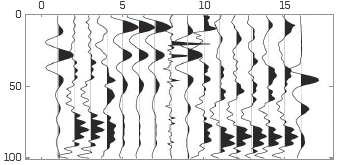Figure 1 Dictionary trained with K-SVD using VSP seismic data; waveforms shown wavelets with different phase.

DENOISING SYNTHETIC VSP DATA

Synthetic data are obtained from a visco-acoustic attenuation model and the source is modelled using a Ricker wavelet with 20 Hz as the dominant frequency a quality factor of 50, a wave velocity of 2000 m/s, and the geophones are placed in separate locations using regular distances. The synthetic signals are contaminated by additive white Gaussian noise with a SNR of 5 dB. For noise reduction, the OMP algorithm is implemented with a window of 100 ms and one sample shifted at a time. Additionally, five coefficients are used for the sparse coding using OMP. Figure 2 shows the seismic traces before being interpolated and denoised. After the interpolating and denoising process, the output traces have a SNR equal to 16 dB, showing an improvement of about 11 dB. We use Fourier bases on the interpolation process to compare reconstructed traces, using OMP with both dictionaries (Fourier bases and dictionaries trained) To determine performance of both approaches, we use matching by correlation between reconstructed traces and the original trace. The correlation of the original trace with the reconstructed trace with OMP using Fourier bases is 0.6170 and 0.6260 using OMP with dictionaries learned (DL).Figure 2 Signal contaminated (zoom 15 traces) with withe Gaussian noise with SNR=5 dB and removed 4 traces.

Figure 3a shows that seismic trace 15 from the synthetic data after the OMP process improve the spectral distribution of the signal and recover the amplitude and phase of the wavelet, showing that relevant content of the trace are preserved. It it also shows that the noise components at low and high frequencies are attenuated.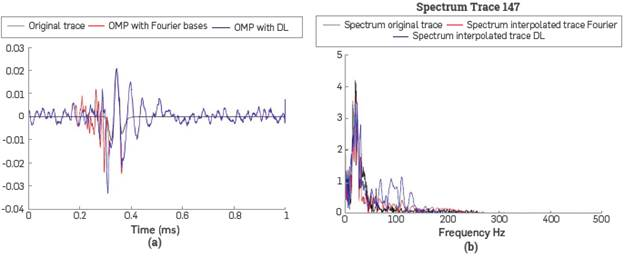Figure 3 (a) Original (black) and interpolated and denoised trace with OMP with Fourier bases (red) and Dictionaries learned (blue). (b) Original (black) and interpolated and denoised (red, blue) amplitude spectrum.

5. RESULTS

ON REAL VSP DATA RESULTS

Improvements in SNR relation and recovery of lost traces is material in VSP processing, considering that quality factor estimation based on logarithmic spectral ratio, centroid frequency-shift, and peak frequency-shift methods are very sensitive to amplitude spectrum distribution.

We design an experiment to evaluate the performance of the OMP algorithm on real data. In this experiment, we removed ten traces from the original data at random locations, as shown in Figure 4 simulating missing traces.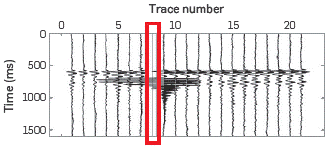Figure 4 (Top) Original traces (zoom over 21 traces) with 10 traces removed.

Assuming that bad traces and traces that first break do not correspond with neighbourhood traces, these are removed in the quality control stage. The application of OMP algorithm must restore these traces by interpolation and attenuate the noise in all the data, as show in Figure 5. Comparison at time and frequency domain between original and recovery traces by OMP is encouraging The correlation between original traces with reconstructed traces with OMP using Fourier bases is 0.6538 and 0.6668 using OMP with dictionaries learned (DL).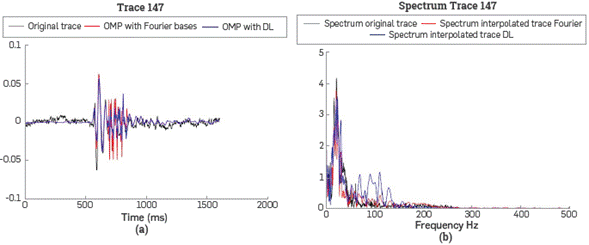Figure 5 (a) Real trace 147 removed (black) and interpolated trace (red), (b) real trace removed (black) and spectrum interpolated trace (red).

NEAR-SURFACE SEISMIC REFRACTION RESULTS

Acquisition in shallow seismic refraction to estimate a P-wave velocity-depth model requires the identification of first-arrival times associated with refracted waves . Correct picking of first arrival is required for the tomography inversion process and it is affected by loss data and low S/N relation traces.

A further problem in land data is the restriction to locate receivers in regular areas; therefore, new data acquisition design based in sparse data is a possible solution. We acquire refraction data to test the performance of OMP algorithm for interpolation of randomly annulled traces.

The experiment acquired 160 different shots with only one trace by shot so as to have sufficient redundancy to build the trained overcomplete dictionary. This dictionary contains main characteristics (shapes) of this kind of signals, which allows representing a wide range of signal phenomena. Figure 6 shows a trained dictionary of 160 atoms (traces) of 100 samples each in time.

To evaluate this method on refraction seismic data, data was acquired using a sample rate of 0.5 ms and 24 different offsets. We remove 6 traces at random positions and used OMP for interpolation of these data.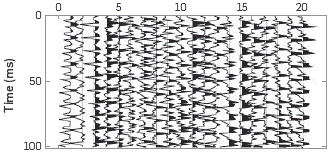Figure 6 Dictionary trained with refraction seismic data.

Figure 7a shows the data with 6 removed traces and the data after the interpolation process (Figure 7b). The correlation of the original traces with reconstructed traces with OMP using dictionaries learned is 0.6513.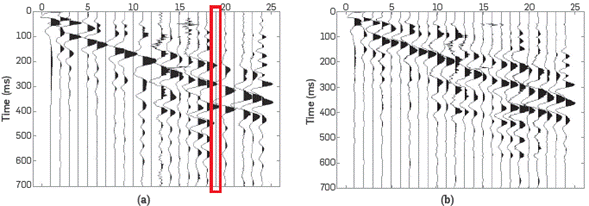Figure 7 (a) Refraction seismic data with 6 traces removed (b) Data with interpolated traces.

Figure 8 shows the original trace and the interpolated trace. The reconstructed traces show the same shape as the original data, and preserve the amplitudes and phases, which produce a very good alignment of the refraction event.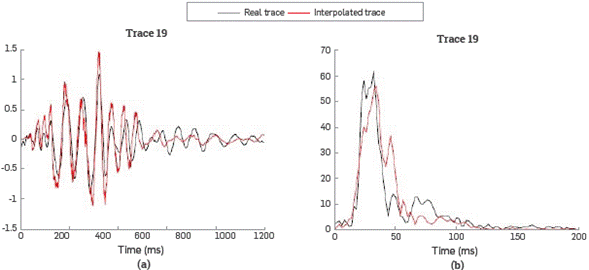Figure 8 (a) original trace 19 removed (black) and interpolated trace (red). (b) original trace 19 removed (black) and interpolated trace (red).

CONCLUSIONS

The main contribution of this work is the implementation and validation with real data of an Orthogonal Matching Pursuit (OMP) algorithm for interpolation and denoising. The advantage of the OMP technique in relation with other interpolation techniques is its robustness against the presence of noise, as it is based on a trained dictionary with redundant data.

Real VSP data test demonstrated that it is possible to accurately recover seismic signals from low signal to noise ratio data eliminating high frequencies through overlapping windows with only a few coefficients from its representation. This result must have an impact on quality factor estimation methods in frequency domain.

This technique works as a reconstruction method that promotes prior knowledge of the signal sparsity. Application to interpolation shows that traces missing randomly on refraction seismic data are recovered with high performance in amplitude and phase.

ACKNOWLEDGEMENTS

We would like to thank Ecopetrol for allowing us to access the data and to publish this work. Rórriulo Sandoval would also like to thank the grant provided by Ecopetrol, Universidad de Pamplona and Colciencias Project 0266-2013.

REFERENCES

 Zhou, Y., Shi, C, Chen, H., Xie, J., Wu, G. and Chen, Y. Spike-Like Blending Noise Attenuation Using Structural Low-Rank Decomposition: IEEE Geoscience and Remote Sensing Letters, 2017, 14 1633-1637. [ Links ]

 Naghizadeh, M and Sacchi, M. f-x adaptive seismic-trace interpolation: Geophysics, 2008, 74 V9-V16. [ Links ]

 Jafarpour, B., Goya I, V ., McLaughlin, D and Freeman, W. Transform-domain sparsity regularizaron for inverse problems in geosciences: Geophysics, 2009, VOL. 74, Na 5 september-October. [ Links ]

 Elad, Michael. (2010). "Sparse and Redundant Representations - From Theory to Applications in Signal and Image Processing." [ Links ]

 Mallat, Stéphane and Zhlfeng Zhang. "Matching cursults with tlme-freguency dictionaries." IEEE Trans. Signal Processing, 1993, 41: 3397-3415. [ Links ]

 Rati, Yagyensh Chandra, Ramln Rezailfar, and Perinkulam Sambamurthy Krlshnaprasad. "Orthogonal matching pursuit: Recursive function approximation with applications to wavelet decomposition." Signals. Systems and Computers. 1993 . 1993 Conference Record of The Twenty-Seventh Asilomar Conference on, 1993, IEEE [ Links ]

 Chen, Scott Shaobing, David L. Donoho, and Michael A. Saunders. "Atomic decomposition by basis pursuit. SIAM review. 2001, 43.1:129-159. [ Links ]

 Kumar, v. and Herrmann, F. Incoherent noise suppression with curvelet-domain sparsity: SEG Houston 2009 International Exposition and Annual Meeting. [ Links ]

 Kim, B., Jeong, S., Byun, J., and Jee, S. Curvelet transform-based POCS in f-k domain: SEG Las Vegas 2012 Annual Meeting. [ Links ]

 Wu ,R., Geng, Y. Ye, L. Preliminary study on Dreamlet based compressive sensing data recovery: SEG Houston 2013 Annual Meeting. [ Links ]

 Rubinstein, R., Zibulevsky, M., and Elad, M. (2008). Efficient implementation of the k-svd algorithm using batch orthogonal matching pursuit: Technical report. [ Links ]

 Zhu, L., Liu, E., and McClellan, J. H.. Seismic data denoising through multiscale and sparsity-promoting dictionary learning: Geophysics, 2015, 80, no. 6. [ Links ]

 Chen, Y., Ma, J., and Fomel, S. Double-sparsity dictionary for seismic noise attenuation: Geophysics , 2016, 81, no. 2, V103-V116. March-April. [ Links ]

 Beckouche, S., and Ma, J. Simultaneous dictionary learning and denoising for seismic data: Geophysics , 2014, 79, no. 3. May-June. [ Links ]

 Pati, N., Pradhan, A., Kanoje, L. and Das T. An approach to Image Compression by using Sparse Approximation Technique: Procedia Computer Science, 2015, 48 (2015) 769 - 775. [ Links ]

 Bhattacharya, G., and Depalle P. Sparse denoising of audio by greedy time-frequency shrinkage: IEEE International Conference on Acoustic, 2014, Speech and Signal Processing (ICASSP). [ Links ]

 Huang, W., Wang, R., Chen, X., Zu, S., and Chen, Y. (2017). Damped sparse representation for seismic random noise attenuation: SEG International Exposition and 87th Annual Meeting. [ Links ]

 Aharon, M., Elad, M., and Bruckstein, A. K-SVD: An algorithm for designing overcomplete dictionaries for sparse representation: IEEE Transactions on signal processing, 2006, 54, no. 11. November. [ Links ]

 Yilmaz, öz. (2015). Engineering Seismology with Applications to Geotechnical Engineering, Society of Exploration Geophysicists. [ Links ]

Received: April 30, 2018; Revised: August 27, 2018; Accepted: October 16, 2018

* email: romusanflo@gmail.comThis is an open-access article distributed under the terms of the Creative Commons Attribution License